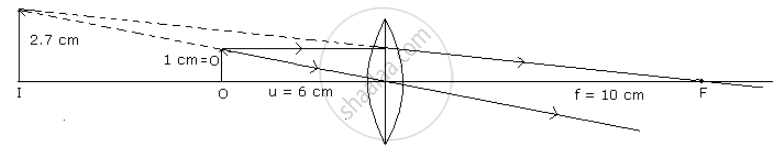Advertisement Remove all ads

# A Pin 2 Cm Long is Placed 12 Cm Away from a Convex Lens a the Principal Axis. If the Focal Length of the Lens is 20 Cm, by Scale Drawing Find the Size of the Image and Its Magnification. - Physics

Numerical

A pin 2 cm long is placed 12 cm away from a convex lens at right angles to the principal axis. If the focal length of the lens is 20 cm, by scale drawing find the size of the image and its magnification.

Advertisement Remove all ads

#### Solution

Given O = 2 cm, u = 12 cm, f = 20 cm

I = ?,   M = ?

Taking the scale of measurement are : 2 cm = 1 cm on the graph.

In the drawing,

O = 1cm, u = 6cm, f = 10 cm

From the drawing,

I = 2.7 cm

⇒ actual image size I = 2.7 x 2 = 5.4 cm

Magnification m = "I"/"O" = 5.4/2 = 2.7 cmConcept: Convex Lens
Is there an error in this question or solution?
Advertisement Remove all ads

#### APPEARS IN

Frank ICSE Class 10 Physics Part 2
Chapter 2 Light
Exercise | Q 1 | Page 113
Advertisement Remove all ads
Advertisement Remove all ads
Share
Notifications

View all notifications

Forgot password?
Course